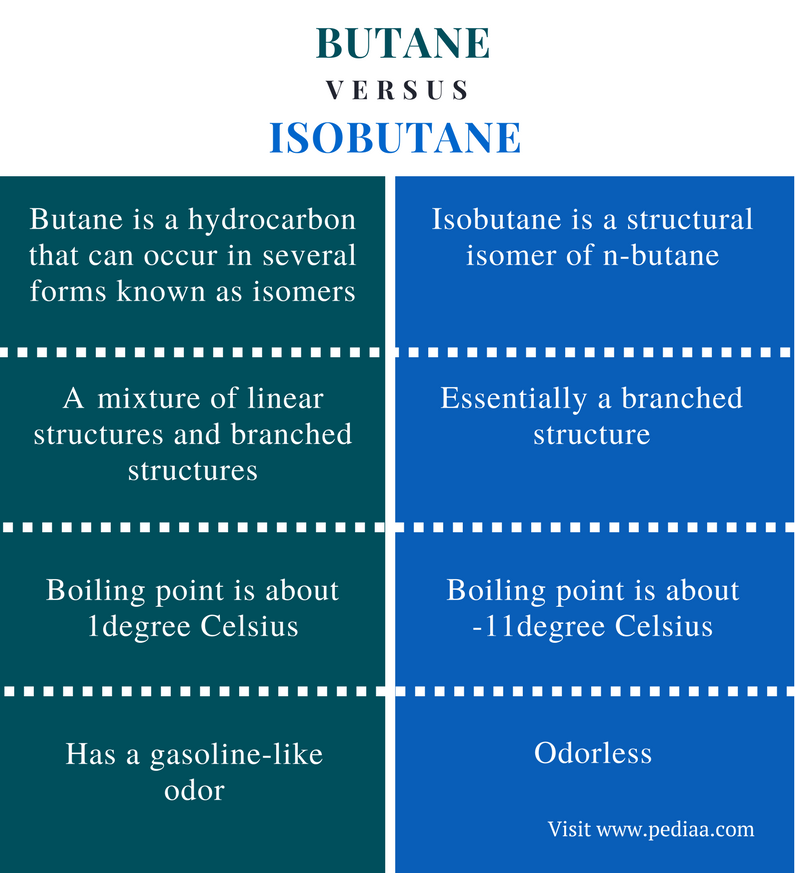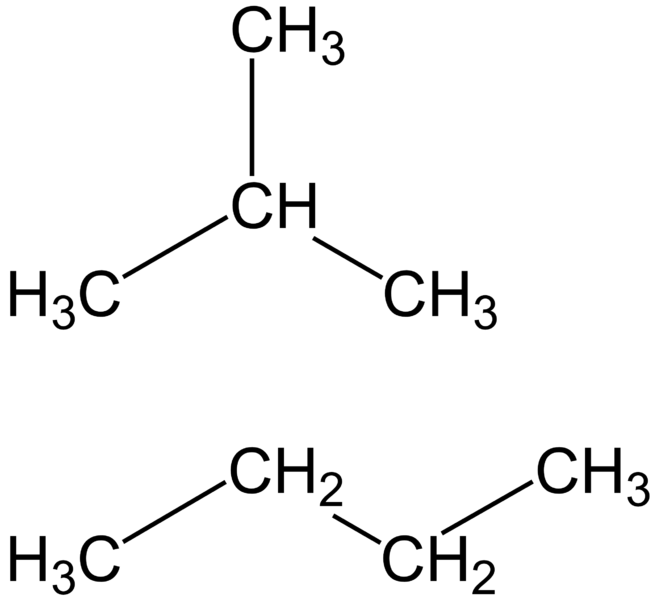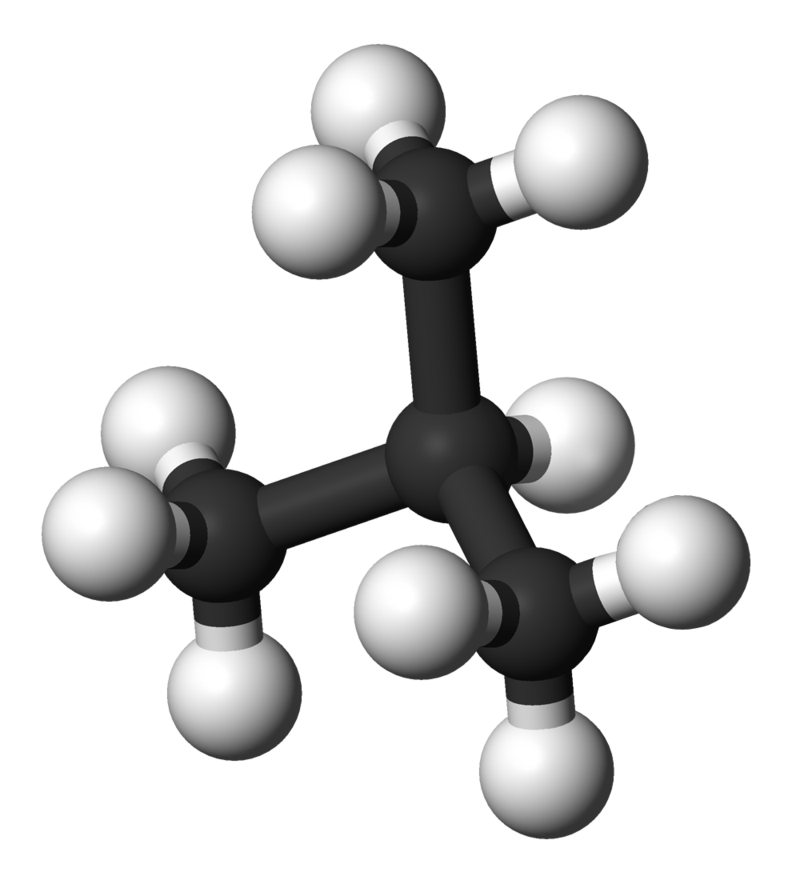# Difference Between Butane and Isobutane

## Main Difference – Butane vs Isobutane

Butane is an organic compound. This molecule can be found in several forms known as isomers. Isobutane is a structural isomer of butane. Both butane and isobutane are gaseous hydrocarbon compounds. They are hydrocarbons because these compounds are composed only of C and H atoms. Both butane and isobutane have the same chemical formula: C4H10. Therefore, they have the same molar mass values. These compounds are flammable and thus, can be used as fuel for burning. The main difference between butane and isobutane is that butane can be either a linear or branched molecule whereas isobutane is essentially a branched molecule.

### Key Areas Covered

1. What is Butane
– Definition, Properties, and Applications
2. What is Isobutane
– Definition, Properties, and Applications
3. What are the Similarities Between Butane and Isobutane
– Outline of Common Features
4. What is the Difference Between Butane and Isobutane
– Comparison of Key Differences

Key Terms: Butane, Fuel, Isobutane, Isomers, Hydrocarbon## What is Butane

Butane is a hydrocarbon that can occur in several forms known as isomers. The chemical formula of butane is C4H10. It is an alkane since there are no double bonds between carbon atoms. The molar mass of butane is about 58 g/mol. At room temperature and atmospheric pressure, butane is a colorless gas that has a gasoline-like odor.

The major source of butane is crude oil. Crude oil has butane as a minor component. Butane is also a byproduct of petroleum refining. It can occur in several isomeric forms. These isomers are called structural isomers. There are two isomers of butane: n-butane and isobutane. n-butane is the linear, straight chain form of butane. Isobutane is the branched structure of butane. The term butane can be used to describe one of these isomers or a mixture of them.Figure 1: Two Isomers of Butane; Isobutane (top) and n-butane (bottom)

Butane can be burnt in the presence of oxygen. This is because butane is highly flammable. If there is enough oxygen, butane undergoes complete combustion. If there isn’t enough oxygen, butane will undergo incomplete combustion. In both cases, combustion produces heat energy.

Butane can be used as an enhancer for the volatilization of gasoline. Therefore, butane is sometimes an additive to gasoline. Butane can also be used as a fuel directly. For example, it can be easily liquefied and be sold for household purposes such as cooking. Since the boiling point of butane is 1oC or less, butane liquid will immediately vaporize at room temperature.

## What is Isobutane

Isobutane is a structural isomer of n-butane. The chemical formula of isobutane is C4H10. The molar mass of isobutane is about 58 g/mol. It is an alkane that has a branched structure. Here, three methyl groups and an H atom is bonded to a central carbon atom. The geometry around the central carbon atom of isobutane is tetrahedral. Isobutane is also a colorless gas at room temperature and atmospheric pressure. Unlike n-butane, it is an odorless gas.Figure 02: Ball and Stick Model of Isobutane

Isobutane has a very low boiling point than that of normal butane. The boiling point of isobutane is about -11oC. The reason for this difference is the branched structure of isobutane. A linear molecule can have more Van Der Waal forces than that of a branched molecule. (The surface area of a linear structure is higher than the surface area of a branched molecule, allowing it to have more Van Der Waal forces between molecules.). The presence of weak or less amount of Van Der Waal forces makes it is easier to separate molecules from each other. Therefore, the boiling point is decreased.

Due to the difference in the spatial arrangement of atoms of isobutane, the chemical and physical properties are different from that of butane. Isobutane can be formed from the isomerization of n-butane. It is a catalytic reaction and is reversible. This isomerization is done through an intermediate called olefins. The efficiency of the process is dependent on the temperature of the system.

Isobutane is used as a refrigerant. Since it has a negligible effect on the ozone layer, isobutane is a good substituent for compounds such as chlorofluorocarbon used in refrigerators.

## Similarities Between Butane and Isobutane

• Butane and Isobutane are hydrocarbon compounds.
• Both have the same chemical formula.
• Both have the same molar mass.
• Both compounds are composed of sp3 hybridized carbon atoms.
• Both are colorless gases at room temperature and atmospheric pressure.

## Difference Between Butane and Isobutane

### Definition

Butane: Butane is a hydrocarbon that can occur in several forms as isomers.

Isobutane: Isobutane is a structural isomer of n-butane.

### Chemical Structure

Butane: Butane is a mixture of linear structures and branched structures.

Isobutane: Isobutane is essentially a branched structure.

### Boiling Point

Butane: The boiling point of butane is about 1oC.

Isobutane: The boiling point of Isobutane is about -11oC.

### Odor

Butane: Butane has a gasoline-like odor.

Isobutane: Isobutane is odorless.

### Conclusion

Butane and isobutane are hydrocarbon compounds that can be found in crude oil as minor constituents. They are small alkane compounds that are called paraffin fraction of petroleum refining. Although they have the same chemical formula and the same molar mass, they are different in several ways. The main difference between butane and isobutane is that butane can be either a linear or branched molecule whereas isobutane is essentially a branched molecule.

##### References:

1. “Butane: Definition, Properties & Formula.” Study.com, Study.com, Available here. Accessed 23 Aug. 2017.
2. “NIST Chemistry WebBook, SRD 69.” Isobutane, National Institute of Standards and Technology, Available here. Accessed 23 Aug. 2017.

##### Image Courtesy

1. “Isobutane-3D-balls” by Ben Mills and Jynto – Derivative of File:Neopentane-3D-balls.png and File:Methane-3D-balls.png. (Public Domain) via Commons Wikimedia
2. “Isobutane-n-butane” ( Public Domain) via Commons Wikimedia